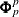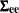### Printed Output

The printed output produced by the STATESPACE procedure includes the following:

1. descriptive statistics, which include the number of observations used, the names of the variables, their means and standard deviations (Std), and the differencing operations used

2. the Akaike information criteria for the sequence of preliminary autoregressive models

3. if the PRINTOUT=LONG option is specified, the sample autocovariance matrices of the input series at various lags

4. if the PRINTOUT=LONG option is specified, the sample autocorrelation matrices of the input series

5. a schematic representation of the autocorrelation matrices, showing the significant autocorrelations

6. if the PRINTOUT=LONG option is specified, the partial autoregressive matrices. (These areas described in the section Preliminary Autoregressive Models.)

7. a schematic representation of the partial autocorrelation matrices, showing the significant partial autocorrelations

8. the Yule-Walker estimates of the autoregressive parameters for the autoregressive model with the minimum AIC

9. if the PRINTOUT=LONG option is specified, the autocovariance matrices of the residuals of the minimum AIC model. This is the sequence of estimated innovation variance matrices for the solutions of the Yule-Walker equations.

10. if the PRINTOUT=LONG option is specified, the autocorrelation matrices of the residuals of the minimum AIC model

11. If the CANCORR option is specified, the canonical correlations analysis for each potential state vector considered in the state vector selection process. This includes the potential state vector, the canonical correlations, the information criterion for the smallest canonical correlation, Bartlett’sstatistic (Chi Square) for the smallest canonical correlation, and the degrees of freedom of Bartlett’s.

12. the components of the chosen state vector

13. the preliminary estimate of the transition matrix,, the input matrix,, and the variance matrix for the innovations,14. if the ITPRINT option is specified, the iteration history of the likelihood maximization. For each iteration, this shows the iteration number, the number of step halvings, the determinant of the innovation variance matrix, the damping factor Lambda, and the values of the parameters.

15. the state vector, printed again to aid interpretation of the following listing ofand16. the final estimate of the transition matrix17. the final estimate of the input matrix18. the final estimate of the variance matrix for the innovations19. a table that lists the estimates of the free parameters inandand their standard errors and t statistics

20. if the COVB option is specified, the covariance matrix of the parameter estimates

21. if the COVB option is specified, the correlation matrix of the parameter estimates

22. if the PRINT option is specified, the forecasts and their standard errors